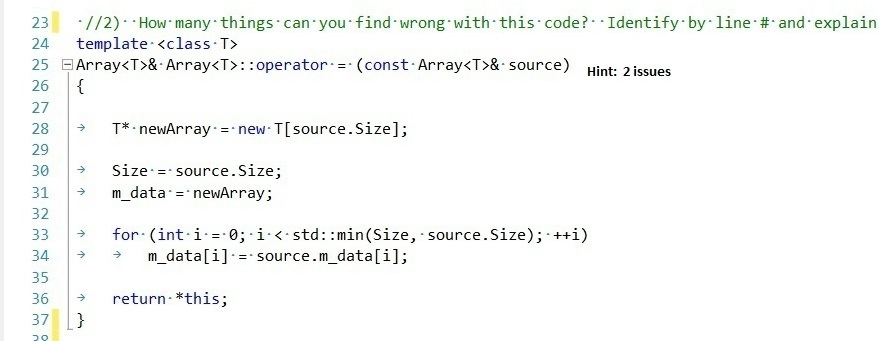C++新手上路，求解这段代码的两个错误及原因3个回答

1、函数一进来应该立马判断 source 和 this 是否相等（因为重载赋值运算符的时候必须要考虑自己给自己赋值的场景），如果二者相等则直接返回*this. 不必再做申请内存和赋值的动作。
2、在 执行 m_data=newArray之前应该要先判断m_data是否为NULL, 如果m_data不是NULL要先讲m_data所指向的内存释放掉，避免内存泄露。

template
Array& Array::operator = (const Array& source)
{
if(this != &source)
{
T* newArray = new T[source.size];
if(m_data != NULL)
{
delete[] m_data;
}
Size = source.size;
m_data = newArray;

``````    for(int i=0; i< std::min(Size, source.size), ++i)
m_data[i] = source.m_data[i];

}
return *this;
``````

}qq_41083830 想了一下觉得应该是这个问题，感谢啦！

`````` template <class T>
Array<T>& Array<T>::operator = (const Array<T>& source)
{
if(this != &source)
{
T* newArray = new T[source.size];
if(m_data != NULL)
{
delete[] m_data;
}
Size = source.size;
m_data = newArray;

for(int i=0; i< std::min(Size, source.size), ++i)
m_data[i] = source.m_data[i];

}
return *this;
}
``````

m_data没有定义
m_data没有释放，造成内存泄漏hnfeng2014 从这个代码片段推测，m_data应该是类Array<T>的成员变量，= 应该是Array<T>中重载的运算符，也就是成员函数，因此m_data不需要在 = 这个函数中定义和释放，而是应该在类Array<T>的构造函数和析构函数中定义和释放。而= 作为成员函数，直接使用 m_data 就好。qq_41083830 回复weixin_43064401: 谢谢啦~weixin_43064401 回复qq_41083830: 应该是qq_41083830 请问new和delete必须成对使用吗？